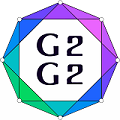#Graphs and Groups, Geometries and GAP (G2G2) Summer School - External Satellite Conference of 8ECM

27 June 2021 to 3 July 2021
Rogla, Slovenia
UTC timezone

## $s$-PD-sets for codes from projective planes $\mathrm{PG}(2,2^h)$, $5 \leq h\leq 9$

Not scheduled
20m
Rogla, Slovenia

#### Rogla, Slovenia

Hotel Planja, Rogla 1, 3214 Zreče, Slovenia
Oral presentation

### Speaker

Nina Mostarac (Department of Mathematics, University of Rijeka)

### Description

In this talk we will describe a construction of $2$-PD-sets of $16$ elements for codes from the Desarguesian projective planes $\mathrm{PG}(2,q)$, where $q=2^h$ and $5\leq h \leq 9$. We will also describe a construction of $3$-PD-sets of $75$ elements for the code from the Desarguesian projective plane $\mathrm{PG}(2,q)$, where $q=2^9$. These $2$-PD-sets and $3$-PD-sets can be used for partial permutation decoding of codes obtained from the Desarguesian projective planes.

### Primary authors

Nina Mostarac (Department of Mathematics, University of Rijeka)